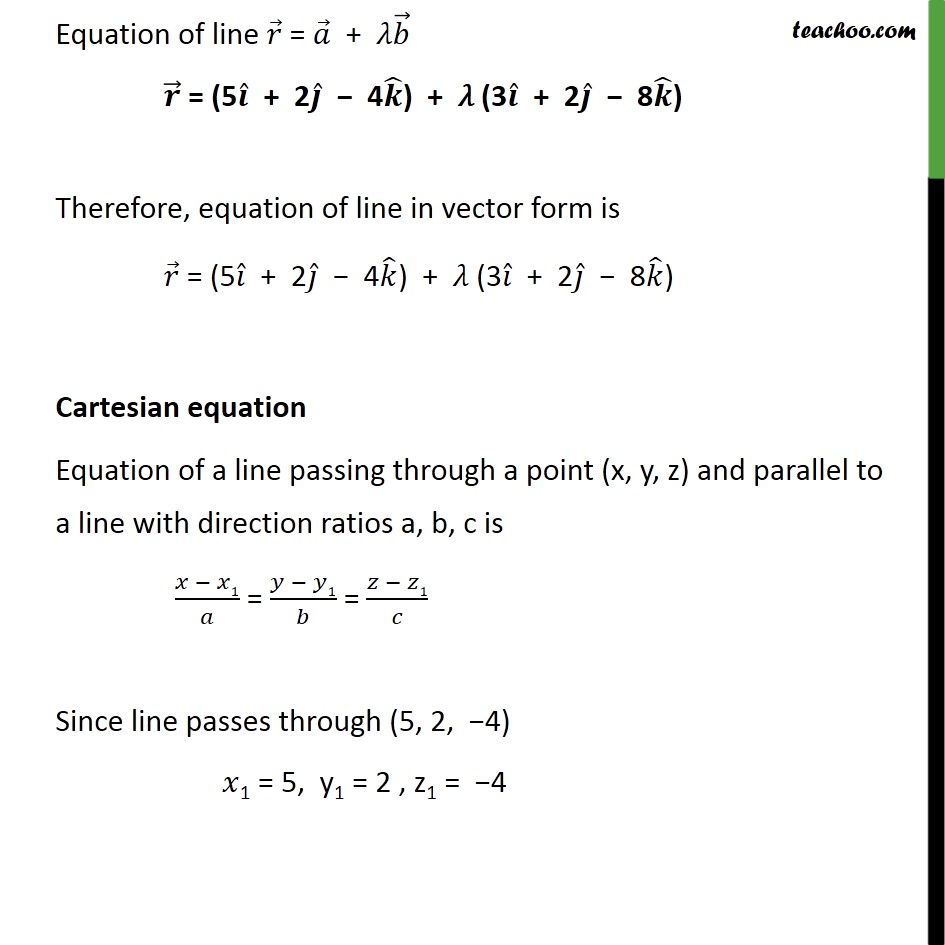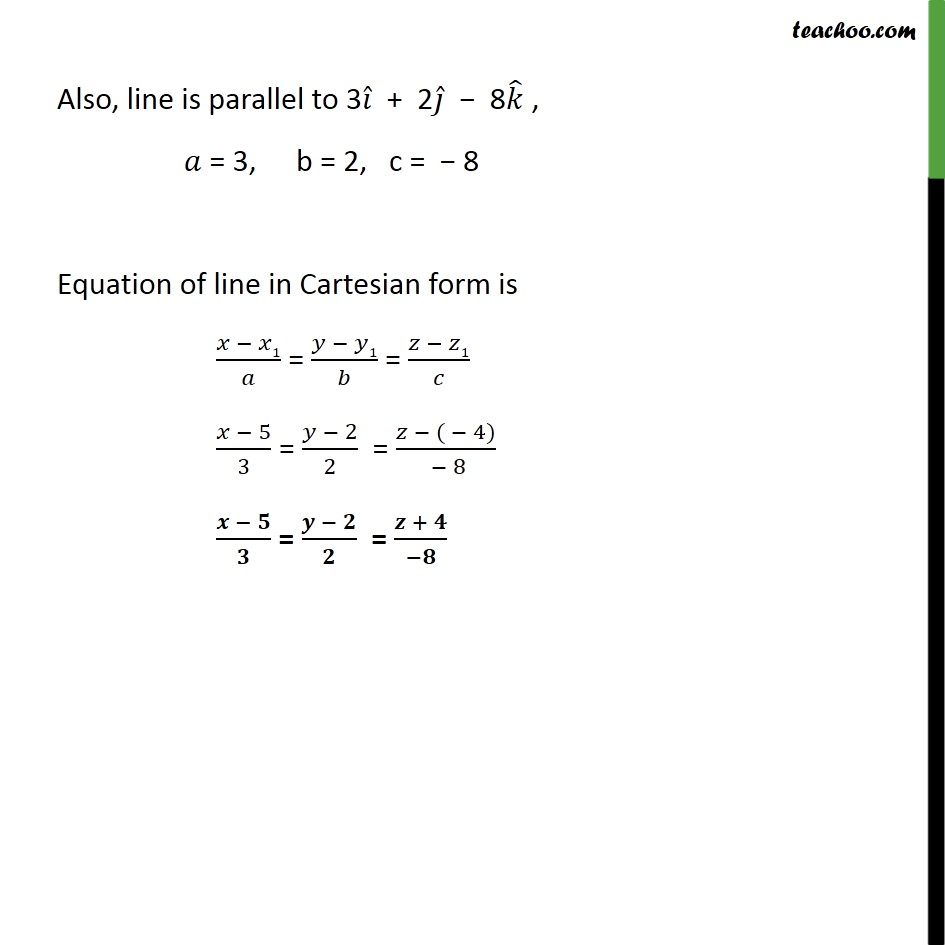1. Chapter 11 Class 12 Three Dimensional Geometry
2. Concept wise
3. Equation of line - given point and //vector

Transcript

Example, 6 Find the vector and the Cartesian equations of the line through the point (5, 2, – 4) and which is parallel to the vector 3𝑖 ̂ + 2𝑗 ̂ – 8𝑘 ̂ . Vector equation Equation of a line passing through a point with position vector 𝑎 ⃗ , and parallel to a vector 𝑏 ⃗ is 𝑟 ⃗ = 𝑎 ⃗ + 𝜆𝑏 ⃗ Since line passes through (5, 2, − 4) 𝑎 ⃗ = 5𝑖 ̂ + 2𝑗 ̂ − 4𝑘 ̂ Since line is parallel to 3𝑖 ̂ + 2𝑗 ̂ − 8𝑘 ̂ 𝑏 ⃗ = 3𝑖 ̂ + 2𝑗 ̂ − 8𝑘 ̂ Equation of line 𝑟 ⃗ = 𝑎 ⃗ + 𝜆𝑏 ⃗ 𝒓 ⃗ = (5𝒊 ̂ + 2𝒋 ̂ − 4𝒌 ̂) + 𝜆 (3𝒊 ̂ + 2𝒋 ̂ − 8𝒌 ̂) Therefore, equation of line in vector form is 𝑟 ⃗ = (5𝑖 ̂ + 2𝑗 ̂ − 4𝑘 ̂) + 𝜆 (3𝑖 ̂ + 2𝑗 ̂ − 8𝑘 ̂) Cartesian equation Equation of a line passing through a point (x, y, z) and parallel to a line with direction ratios a, b, c is (𝑥 − 𝑥1)/𝑎 = (𝑦 − 𝑦1)/𝑏 = (𝑧 − 𝑧1)/𝑐 Since line passes through (5, 2, −4) 𝑥1 = 5, y1 = 2 , z1 = −4 Also, line is parallel to 3𝑖 ̂ + 2𝑗 ̂ − 8𝑘 ̂ , 𝑎 = 3, b = 2, c = − 8 Equation of line in Cartesian form is (𝑥 − 𝑥1)/𝑎 = (𝑦 − 𝑦1)/𝑏 = (𝑧 − 𝑧1)/𝑐 (𝑥 − 5)/3 = (𝑦 − 2)/2 = (𝑧 − ( − 4))/( − 8) (𝒙 − 𝟓)/𝟑 = (𝒚 − 𝟐)/𝟐 = (𝒛 + 𝟒)/(−𝟖)

Equation of line - given point and //vector

About the AuthorDavneet Singh
Davneet Singh is a graduate from Indian Institute of Technology, Kanpur. He has been teaching from the past 9 years. He provides courses for Maths and Science at Teachoo.